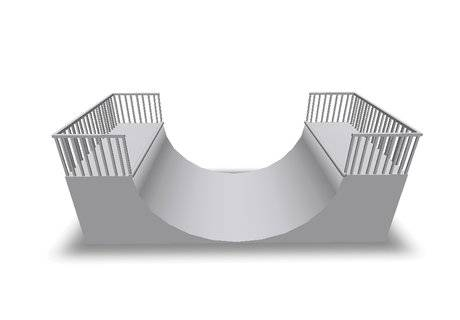# Elastic Collision in Two Reference Frames

Bonnie

## Homework Statement

1. Two skateboarders start from rest on opposite sides of a ramp like the one in the image, roll down and collide elastically on the level part of the ramp. The masses of the skateboarders are m1 = 48 kg and m2 = 55 kg and they both start from the height h = 4.70m. Ignoring any friction, calculate the maximum heights that each skateboarder reaches after the collision
(a) by performing the collision calculation in the laboratory frame
(b) by performing the collision calculation in the centre of momentum frame## Homework Equations

Conservation of energy/momentum
1/2mv2 = mgh
mvi = mvf

## The Attempt at a Solution

I worked out the velocity and momentum of each skater at the instant before they collide, thinking that I could then use normal conservation of momentum and energy to work out the same values for afterward, but as I reached this point I realized that I do not know how to proceed because I have no final values to substitute into the post-collision equation, so do not know how to proceed from here. In the lab frame I have calculated the pre-collision momentum of skater 1 to be 460.8kgm/s, and skater 2 to be 528kgm/s